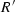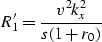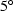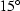Next: Numerical Examples Up: Theory review Previous: Redefining R

## Controlling the angle

It is possible to perform a dip filter with our phase-shift migration algorithm; because we calculatewith the Muir recursion (4,8), we can use the recursion starting with R0=r0s, in order to have control on the desire dip.(14)

where r0 defines the cosine of the angle that starts the Muir recurrence, oftenor; following the recursion (8) we find(15)

and subsequently:(16)

The expressions (14), (15), and (16) are the relations for a,, anddip migration in the Fourier-domain, respectively.Next: Numerical Examples Up: Theory review Previous: Redefining R
Stanford Exploration Project
4/29/2001